# AP Environmental Science : Efficiency

## Example Questions

### Example Question #1 : Energy Conservation

Gasoline has an energy density of roughly 122.8 megajoules per gallon. A car company has produced a new economy car that has a fuel economy of 35 miles per gallon, and 0.877 MJ is exerted per mile to power the forward motion of the vehicle. What is the percent fuel efficiency of the car?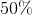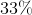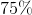Explanation:

The fuel efficiency of the vehicle can be calculated as such: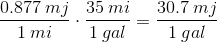This equation gives us the energy converted to forward motion per gallon of gas. We can use this value to determine the percent of total fuel energy that is devoted to forward motion: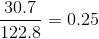From these calculations, we can determine that 25% of the energy from a gallon of gas is converted to actual forward motion of the vehicle, giving us a fuel efficiency value of 25%.

### Example Question #2 : Efficiency

What are net-zero-energy buildings?

Buildings that yield as much or more energy than they need.

Building that produce energy.

Building that as insulated in such a way that they do not require a heating source. They are heated through light bulbs, humans, etc.

Building installed with solar panels.

Buildings whose windows are superinsulated.